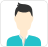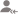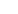true

Learn MATLAB from the Best Tutors

•Affordable fees
•1-1 or Group class
•Flexible Timings
•Verified Tutors

Search in# Learn MATLAB with Free Lessons & Tips

Post a Lesson

All

All

Lessons

Discussion

Lesson Posted on 08/11/2018 Learn MATLABManibhushan Kumar

I have 15 years of experience in Maths and Stats.I have started teaching 1 year after completing my graduate...

A trigonometric identity is given by: cos^2(x/2)=(tanx+sinx)/2tanx We would calculate each side of the equation, substituting.x=pi/2 sol. >> x=pi/5; LHS=cos(x/2)^2 LHS = 0.9045 RHS=(tan(x)+sin(x))/(2*tan(x)) RHS = 0.9045 read more

A trigonometric identity is given by: cos^2(x/2)=(tanx+sinx)/2tanx

We would calculate each side of the equation, substituting.x=pi/2

sol.

>> x=pi/5;

LHS=cos(x/2)^2

LHS =

0.9045

RHS=(tan(x)+sin(x))/(2*tan(x))

RHS =

0.9045

Dislike Bookmark

Lesson Posted on 07/11/2018 Learn MATLABManibhushan Kumar

I have 15 years of experience in Maths and Stats.I have started teaching 1 year after completing my graduate...

Dislike BookmarkVenkatasubramanian Krishnamoorthy

I would say MATLAB is a better option.
Dislike Bookmark

Learn MATLAB from the Best Tutors

•Affordable fees
•Flexible Timings
•Choose between 1-1 and Group class
•Verified TutorsKeerti T.

Define axis in your code. x axis starting from 0 to 5 or 6 and y axis -1 to 11 or 12. Then, You will be able to see x(1)=3. axis()
Dislike BookmarkVenkatasubramanian Krishnamoorthy

Simulink, which is a part of MATLAB has robotic toolbox with which you can build your own models and test its kinematics and dynamics. Basically it allows you to design, simulate and test your designs. You can start by building a simple two-link robotic arm, speciify its degree of freedom (DOF) simulate... read more

Simulink, which is a part of MATLAB has robotic toolbox with which you can build your own models and test its kinematics and dynamics. Basically it allows you to design, simulate and test your designs.

You can start by building a simple two-link robotic arm, speciify its degree of freedom (DOF) simulate and see for yourself how it works.

Dislike BookmarkVenkatasubramanian Krishnamoorthy

Each tool has its pros and cons. It totally depends on what kind of analysis you want to do and in what format do you have the data needed for the analysis. Generally speaking one can go with R, MATLAB or Statistics not necessarily in that order.
Dislike Bookmark

Learn MATLAB from the Best Tutors

•Affordable fees
•Flexible Timings
•Choose between 1-1 and Group class
•Verified TutorsVenkatasubramanian Krishnamoorthy

My suggestion would be to go for SIMULINK (a part of MATLAB) where it gives you lot of model building options by just dragging and dropping different input/ouput/connecting systems e.g: robotics toolbox.
Dislike BookmarkAshok Rai

Computer Wizard

due to its fast mayhematical computation
Dislike BookmarkVenkatasubramanian Krishnamoorthy

Moving average is nothing but a FIR filter. You can use fir1, fir2 commands. For further info on how to use the commands search in mathworks website or google it.
Dislike Bookmark

Learn MATLAB from the Best Tutors

•Affordable fees
•Flexible Timings
•Choose between 1-1 and Group class
•Verified TutorsBEST Technical Trainer & Freelancer

Kindly go through my profile
Dislike Bookmark

UrbanPro.com helps you to connect with the best MATLAB Training in India. Post Your Requirement today and get connected.

Overview

Questions 820

Total Shares6,518 Followers

## MATLAB in:

x

X

### Looking for MATLAB Classes?

The best tutors for MATLAB Classes are on UrbanPro

• Select the best Tutor
• Book & Attend a Free Demo
• Pay and start Learning### Learn MATLAB with the Best Tutors

The best Tutors for MATLAB Classes are on UrbanPro